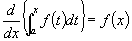IV. INTEGRATION OF TRIGONOMETRIC FUNCTIONS

Integrating the following with respect to x

1.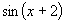2.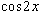3.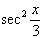4.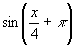5.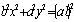6.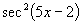7.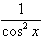8.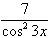9.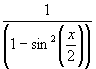10.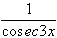11.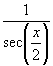12.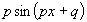13.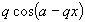14.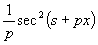15.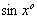16.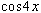17.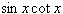Find the following integrals:

18.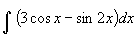19.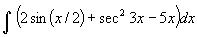20.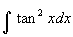21.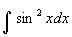22.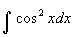23.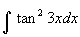24.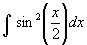25.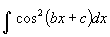26.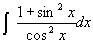27.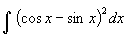28.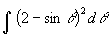29. (a) Show that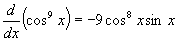, and hence find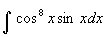(b) Show that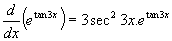, and hence find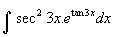(c) Prove that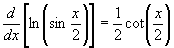, and hence find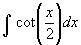(d) Prove by differentiation that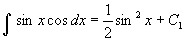=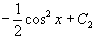or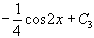. Show that each of the primitive functions differ only by a constant.

30. Find the following integrals by using the substitution method:

(a)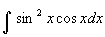(b)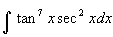(c)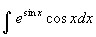(d)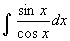(e)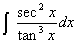(f)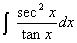(g)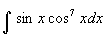(h)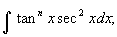(i)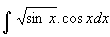(j)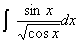(k)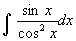(l)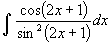(m)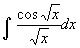(n)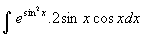(o)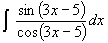(p)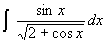(q)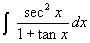31. Determine the value of the following integrals:

(a)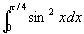(b)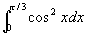(c)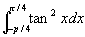(d)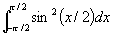(e)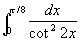(f)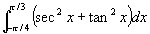(g)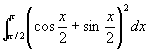(h)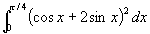32. (a) Find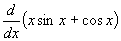hence find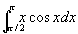.

(b) Show that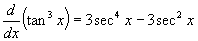hence find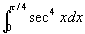(c) Show that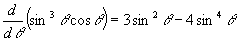hence find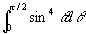(d) Differentiate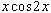and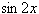; make use of these results to find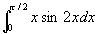.

(e) Show that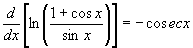and hence find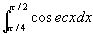.

(f) Show that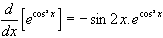, and hence find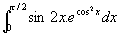(g) Differentitate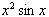and hence find.

33. (a) For what values of a does (i)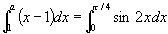and

(ii)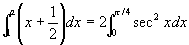(b) Find (i)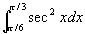(ii)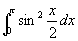(c) Prove that (i)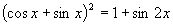(ii)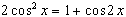(iii)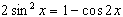, hence find the following integrals: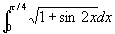,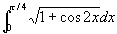,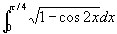.

(d) Show that (i)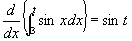(ii)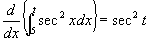(iii)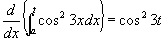(iv)